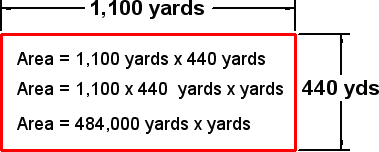Select Page

In tip #148 we explained that the integral is an area or “area under the curve” as we say in class. At a recent training event Rajen was trying to answer a couple of tough remaining questions.

1. “Why are the units shown as psi-seconds?” (or bar-seconds)Just as we multiply length by width we also multiply the units: yards x yards. Though this is normally abbreviated “square yards” but can also be written “yards-yards”.

Now a cavity pressure curve is not bounded by lengths but by pressure and time thus:So when we multiply our little rectangles to compute the area the rectangles have a height in pressure in “psi” (or bar) and width in time in “seconds”. Thus, just as you multiply yards x yards and state it “yards–yards” you do the same here, multiplying the pressure units times time units, psi x seconds and stating it “psi-seconds”.

Note that this is not a division as would be the case in a slope of so many psi per second or “psi / second”. The dash indicates multiplication.
2. Why are the numbers so large?

The “2 seconds” is absurdly long for accuracy. We use it here just for easy illustration of the arithmetic. The eDART actually uses slices in the range of 1/500th of a second (0.002).